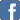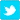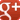Fun With Numbers

Posted on the 20 September 2019 by Markwadsworth @Mark_Wadsworth

The Lass and I were brainstorming yesterday, and between us, came up with this sequence/pattern, where x,y are positive integers.
I am using the European convention of using a full stop to denote 'multiply', so "x^3.y" means "x-cubed times y" (to avoid confusion with "x^3y" which could mean "x to the power of three y").
x^1 - y^1 = (x - y)
x^2 - y^2 = (x - y)(x + y)
x^3 - y^3 = (x - y)(x^2 + x.y + y^2)
x^4 - y^4 = (x - y)(x^3 + x^.2y + x.y^2 + y^3)
We didn't work out x^5 - y^5 the long way, but my initial assumption is...
x^5 - y^5 =(x - y)(x^4 + x^3.y + x^2.y^2 + x.y^3 + y^4)